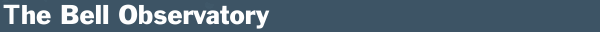General Relativity/Atomic Physics Papers

General Relativity Papers

This page provides links to papers on how to simulate relativistic orbits, in the domains of both the very big and the very small. The first general relativity paper, entitled "A Numerical Solution of the Relativistic Kepler Problem," describes the algorithms necessary to implement Schwarzschild-type orbits. The second general relativity paper presents the results for the more complicated Kerr orbits. The Schwarzschild paper can be accessed by clicking on this link.

This link will take you to the second general relativity article previously made available by Computers in Physics, published by the American Insitute of Physics, on a numerical application of the General Theory of Relativity, entititled "Simulating Relativistic Orbits about a Black Hole." The paper describes how to numerically simulate Kerr orbits. There, you can download the text and figures of the article in Postscript, in Adobe Acrobat's PDF format, or in a TeX ASCII text file.

To download an ASCII file containing the FORTRAN source code of an orbit simulation , please click on keppap5.for. For a detailed explanation of the computer application GR equations used here, please see the following links. The "laboratory" paradigm of Weinberg has been used, and it is best to start with his description of the connections between the Euclidean-Cartesian laboratory coordinate frame used here, and the Kerr frame used to model the acceleration field permeating the laboratory, as generated by the central body. These mathematical developments can be seen here. Next, the equations of motion (3.2.3) can be written in matrix algebra for computer implementation (all tensor algebra is always implemented using matrix algebra on computers). The matrix algebra representation can be seen here. To see how complicated/nonlinear the full set of Kerr equations of motion are, the partial derivatives used are given in complete detail here.

The following link will take you to an atomic theory paper entitled "Non-Euclidean Electromagnetic Kerr Model for Hydrogen." This paper presents my views on how the spherical and aspherical orbitals of a hydrogen atom can be modeled using quantized electromagnetic versions of Schwarzschild and Kerr differential geometries.